Anzeige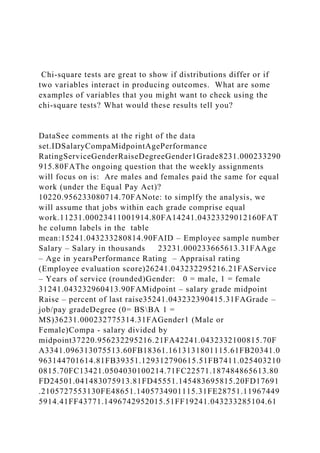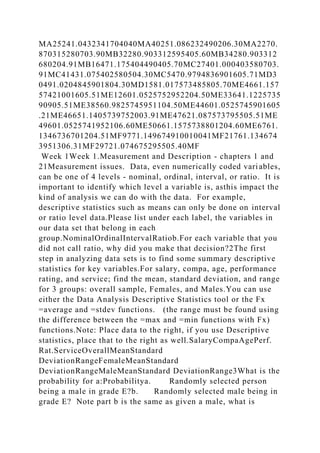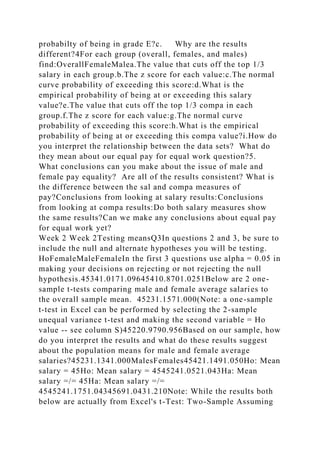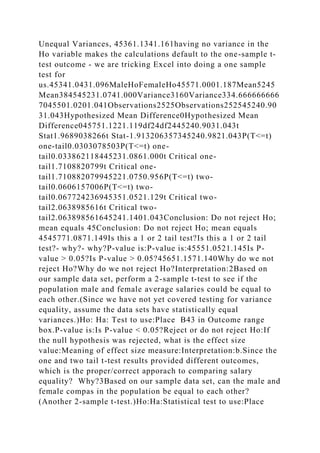Anzeige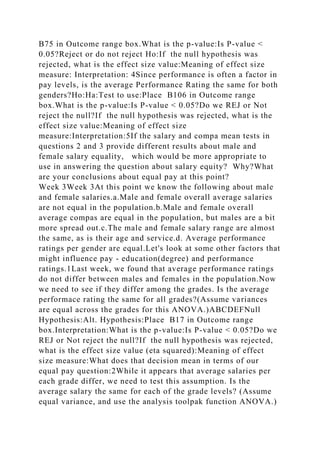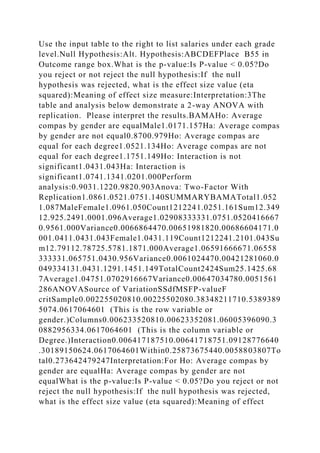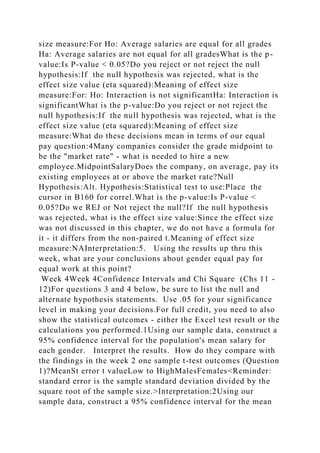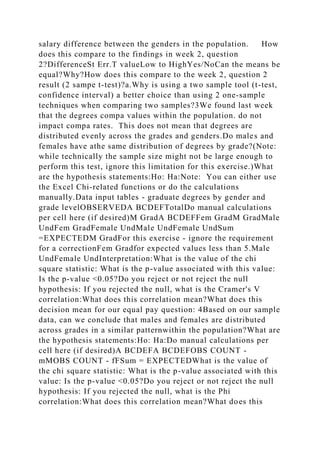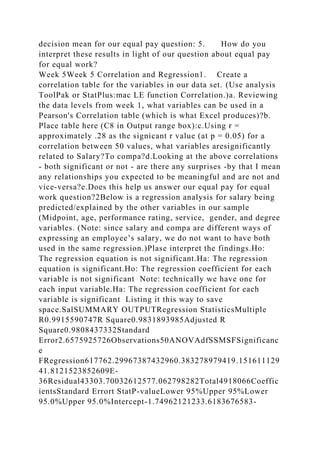Anzeige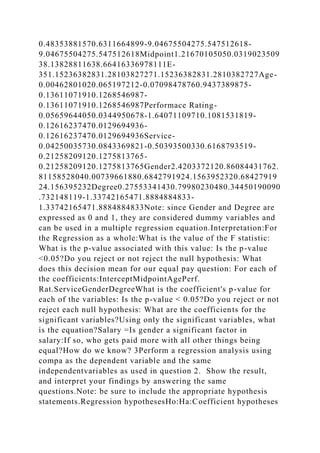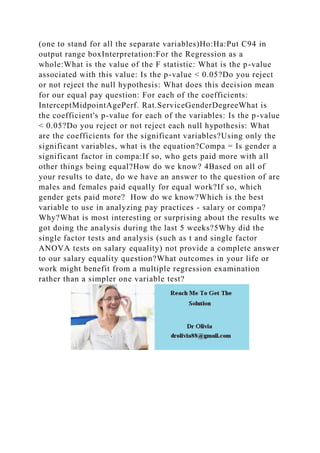Nächste SlideShare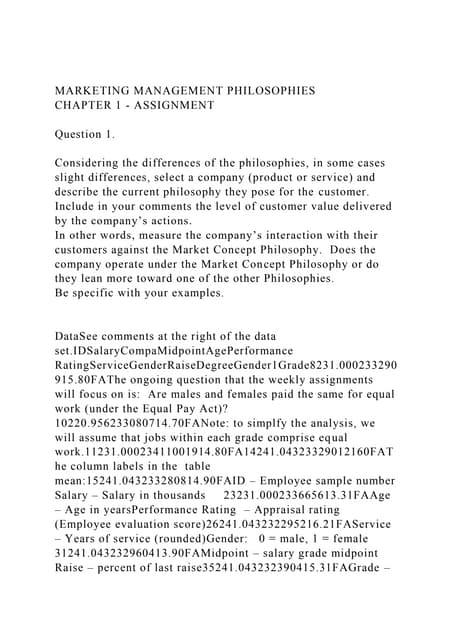MARKETING MANAGEMENT PHILOSOPHIESCHAPTER 1 - ASSIGNMENTQuest.docx
1 von 11
Anzeige

### Chi-square tests are great to show if distributions differ or i.docx

1. Chi-square tests are great to show if distributions differ or if two variables interact in producing outcomes. What are some examples of variables that you might want to check using the chi-square tests? What would these results tell you? DataSee comments at the right of the data set.IDSalaryCompaMidpointAgePerformance RatingServiceGenderRaiseDegreeGender1Grade8231.000233290 915.80FAThe ongoing question that the weekly assignments will focus on is: Are males and females paid the same for equal work (under the Equal Pay Act)? 10220.956233080714.70FANote: to simplfy the analysis, we will assume that jobs within each grade comprise equal work.11231.00023411001914.80FA14241.04323329012160FAT he column labels in the table mean:15241.043233280814.90FAID – Employee sample number Salary – Salary in thousands 23231.000233665613.31FAAge – Age in yearsPerformance Rating – Appraisal rating (Employee evaluation score)26241.043232295216.21FAService – Years of service (rounded)Gender: 0 = male, 1 = female 31241.043232960413.90FAMidpoint – salary grade midpoint Raise – percent of last raise35241.043232390415.31FAGrade – job/pay gradeDegree (0= BSBA 1 = MS)36231.000232775314.31FAGender1 (Male or Female)Compa - salary divided by midpoint37220.956232295216.21FA42241.0432332100815.70F A3341.096313075513.60FB18361.1613131801115.61FB20341.0 963144701614.81FB39351.129312790615.51FB7411.025403210 0815.70FC13421.0504030100214.71FC22571.187484865613.80 FD24501.041483075913.81FD45551.145483695815.20FD17691 .2105727553130FE48651.1405734901115.31FE28751.11967449 5914.41FF43771.1496742952015.51FF19241.043233285104.61
2. MA25241.0432341704040MA40251.086232490206.30MA2270. 870315280703.90MB32280.903312595405.60MB34280.903312 680204.91MB16471.175404490405.70MC27401.000403580703. 91MC41431.075402580504.30MC5470.9794836901605.71MD3 0491.0204845901804.30MD1581.017573485805.70ME4661.157 57421001605.51ME12601.0525752952204.50ME33641.1225735 90905.51ME38560.9825745951104.50ME44601.0525745901605 .21ME46651.1405739752003.91ME47621.087573795505.51ME 49601.0525741952106.60ME50661.1575738801204.60ME6761. 1346736701204.51MF9771.149674910010041MF21761.134674 3951306.31MF29721.074675295505.40MF Week 1Week 1.Measurement and Description - chapters 1 and 21Measurement issues. Data, even numerically coded variables, can be one of 4 levels - nominal, ordinal, interval, or ratio. It is important to identify which level a variable is, asthis impact the kind of analysis we can do with the data. For example, descriptive statistics such as means can only be done on interval or ratio level data.Please list under each label, the variables in our data set that belong in each group.NominalOrdinalIntervalRatiob.For each variable that you did not call ratio, why did you make that decision?2The first step in analyzing data sets is to find some summary descriptive statistics for key variables.For salary, compa, age, performance rating, and service; find the mean, standard deviation, and range for 3 groups: overall sample, Females, and Males.You can use either the Data Analysis Descriptive Statistics tool or the Fx =average and =stdev functions. (the range must be found using the difference between the =max and =min functions with Fx) functions.Note: Place data to the right, if you use Descriptive statistics, place that to the right as well.SalaryCompaAgePerf. Rat.ServiceOverallMeanStandard DeviationRangeFemaleMeanStandard DeviationRangeMaleMeanStandard DeviationRange3What is the probability for a:Probabilitya. Randomly selected person being a male in grade E?b. Randomly selected male being in grade E? Note part b is the same as given a male, what is
3. probabilty of being in grade E?c. Why are the results different?4For each group (overall, females, and males) find:OverallFemaleMalea.The value that cuts off the top 1/3 salary in each group.b.The z score for each value:c.The normal curve probability of exceeding this score:d.What is the empirical probability of being at or exceeding this salary value?e.The value that cuts off the top 1/3 compa in each group.f.The z score for each value:g.The normal curve probability of exceeding this score:h.What is the empirical probability of being at or exceeding this compa value?i.How do you interpret the relationship between the data sets? What do they mean about our equal pay for equal work question?5. What conclusions can you make about the issue of male and female pay equality? Are all of the results consistent? What is the difference between the sal and compa measures of pay?Conclusions from looking at salary results:Conclusions from looking at compa results:Do both salary measures show the same results?Can we make any conclusions about equal pay for equal work yet? Week 2 Week 2Testing meansQ3In questions 2 and 3, be sure to include the null and alternate hypotheses you will be testing. HoFemaleMaleFemaleIn the first 3 questions use alpha = 0.05 in making your decisions on rejecting or not rejecting the null hypothesis.45341.0171.09645410.8701.0251Below are 2 one- sample t-tests comparing male and female average salaries to the overall sample mean. 45231.1571.000(Note: a one-sample t-test in Excel can be performed by selecting the 2-sample unequal variance t-test and making the second variable = Ho value -- see column S)45220.9790.956Based on our sample, how do you interpret the results and what do these results suggest about the population means for male and female average salaries?45231.1341.000MalesFemales45421.1491.050Ho: Mean salary = 45Ho: Mean salary = 4545241.0521.043Ha: Mean salary =/= 45Ha: Mean salary =/= 4545241.1751.04345691.0431.210Note: While the results both below are actually from Excel's t-Test: Two-Sample Assuming
4. Unequal Variances, 45361.1341.161having no variance in the Ho variable makes the calculations default to the one-sample t- test outcome - we are tricking Excel into doing a one sample test for us.45341.0431.096MaleHoFemaleHo45571.0001.187Mean5245 Mean384545231.0741.000Variance3160Variance334.666666666 7045501.0201.041Observations2525Observations252545240.90 31.043Hypothesized Mean Difference0Hypothesized Mean Difference045751.1221.119df24df2445240.9031.043t Stat1.9689038266t Stat-1.913206357345240.9821.043P(T<=t) one-tail0.0303078503P(T<=t) one- tail0.033862118445231.0861.000t Critical one- tail1.7108820799t Critical one- tail1.710882079945221.0750.956P(T<=t) two- tail0.0606157006P(T<=t) two- tail0.067724236945351.0521.129t Critical two- tail2.0638985616t Critical two- tail2.063898561645241.1401.043Conclusion: Do not reject Ho; mean equals 45Conclusion: Do not reject Ho; mean equals 4545771.0871.149Is this a 1 or 2 tail test?Is this a 1 or 2 tail test?- why?- why?P-value is:P-value is:45551.0521.145Is P- value > 0.05?Is P-value > 0.05?45651.1571.140Why do we not reject Ho?Why do we not reject Ho?Interpretation:2Based on our sample data set, perform a 2-sample t-test to see if the population male and female average salaries could be equal to each other.(Since we have not yet covered testing for variance equality, assume the data sets have statistically equal variances.)Ho: Ha: Test to use:Place B43 in Outcome range box.P-value is:Is P-value < 0.05?Reject or do not reject Ho:If the null hypothesis was rejected, what is the effect size value:Meaning of effect size measure:Interpretation:b.Since the one and two tail t-test results provided different outcomes, which is the proper/correct apporach to comparing salary equality? Why?3Based on our sample data set, can the male and female compas in the population be equal to each other? (Another 2-sample t-test.)Ho:Ha:Statistical test to use:Place